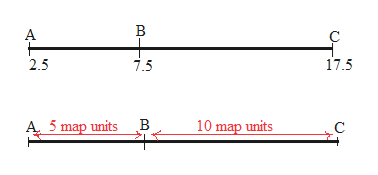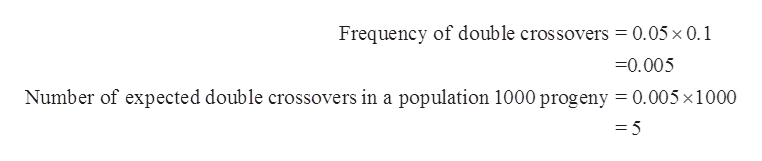# Genes A, B, and C lie at map positions 2.5, 7.5, and 17.5, respectively. In a three-point test cross, you observed 2 double crossovers in a total of 1000 progeny. What is the interference in this region?

Question
37 views

Genes A, B, and C lie at map positions 2.5, 7.5, and 17.5, respectively. In a three-point test cross, you observed 2 double crossovers in a total of 1000 progeny. What is the interference in this region?

check_circle

Step 1

The measure of genetic linkage is termed as recombination frequency, which is used in the construction of a genetic linkage map. Recombination frequency is depicted by Ɵ (theta) and calculates the frequency by which a single chromosomal crossover occurs between two genes at the time of meiosis. Its formula is:

Step 2

Map distance between gene A is 2.5 map units.

Map distance between gene B is 7.5 map units.

Map distance between gene C is 17.5 map units.

Therefore, the distance between A and B is 5 map units.

The distance between B and C is 10 map units.

The representation for above is shown below:help_outlineImage TranscriptioncloseВ А с 17.5 7.5 2.5 В 5 map units 10 map units A C fullscreen
Step 3

It is known that 1 map unit is equal to 1% recombination frequency.

Therefore, recombination frequency between A and...help_outlineImage TranscriptioncloseFrequency of double crossovers = 0.05x 0.1 -0.005 Number of expected double crossovers in a population 1000 progeny = 0.005 x1000 = 5 fullscreen

### Want to see the full answer?

See Solution

#### Want to see this answer and more?

Solutions are written by subject experts who are available 24/7. Questions are typically answered within 1 hour.*

See Solution
*Response times may vary by subject and question.
Tagged in